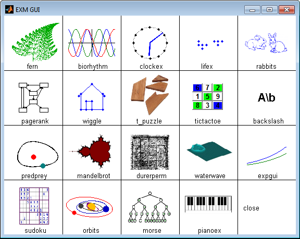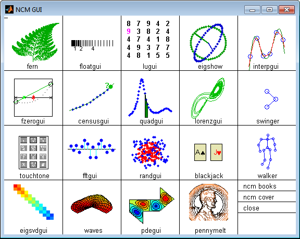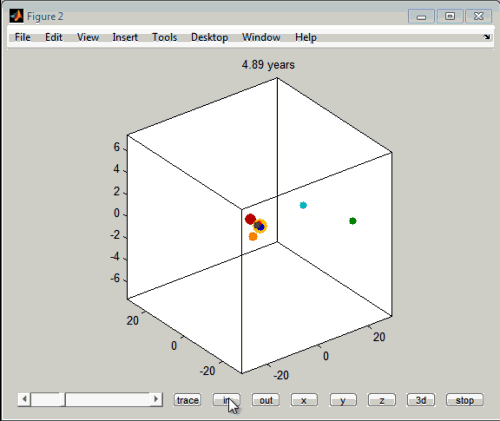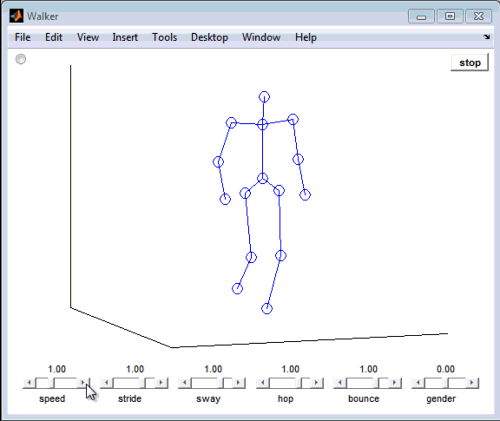# Cleve’s e-Books

Jiro's pick this week is "Experiments with MATLAB" and "Numerical Computing with MATLAB" by Cleve Moler. Some of you may know Cleve... :) Today, I'd like to highlight two things:If you didn't know, Cleve has written two electronic books on using MATLAB for mathematics. The "Numerical Computing with MATLAB" book is also available in traditional print format. They come with a wealth of MATLAB examples, some of which are suitable for high school students (in "Experiments with MATLAB"). You were able to download the example code from their web pages before, but now, if you have R2012b, you can install the examples as an App with a single click! Here are a couple of my favorites from his examples.

Experiments with MATLAB - OrbitsNumerical Computing with MATLAB - Walker